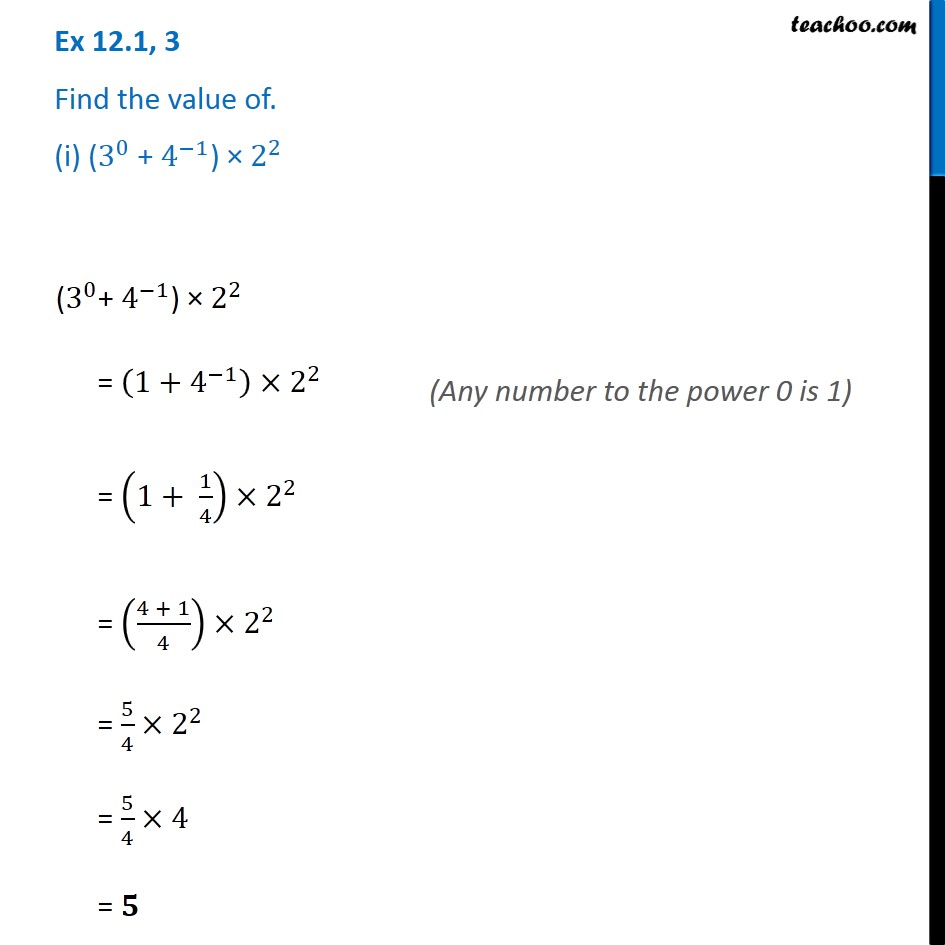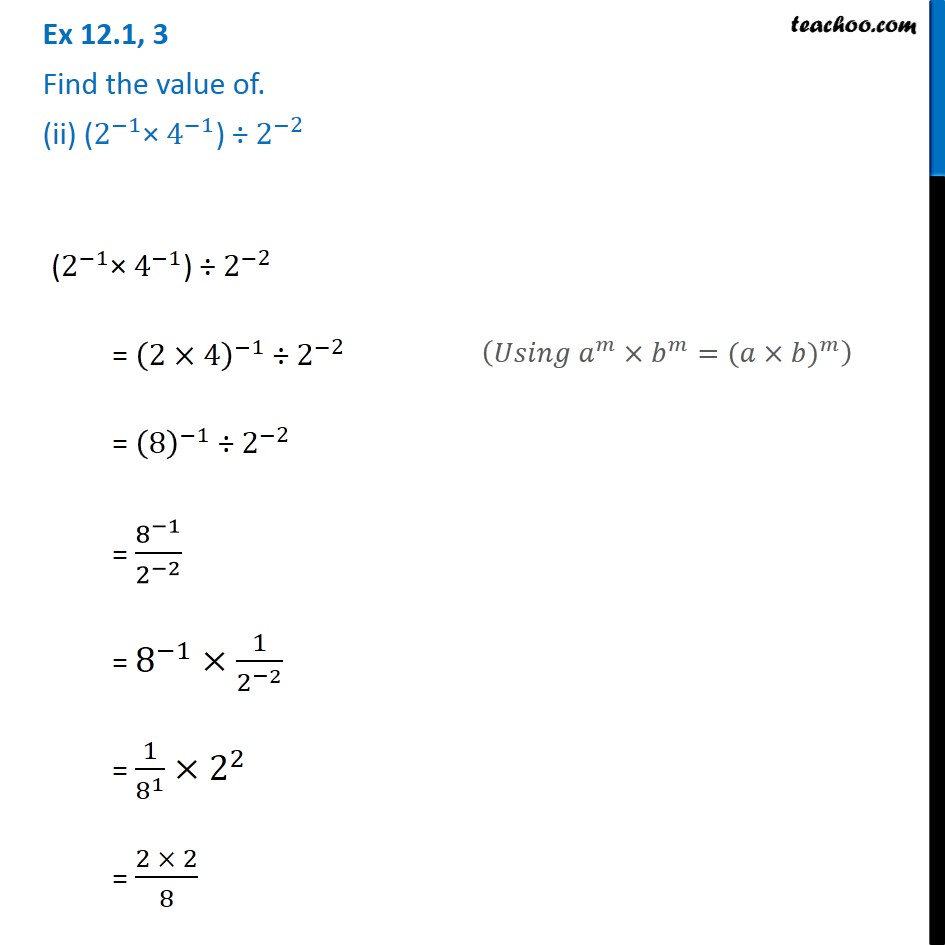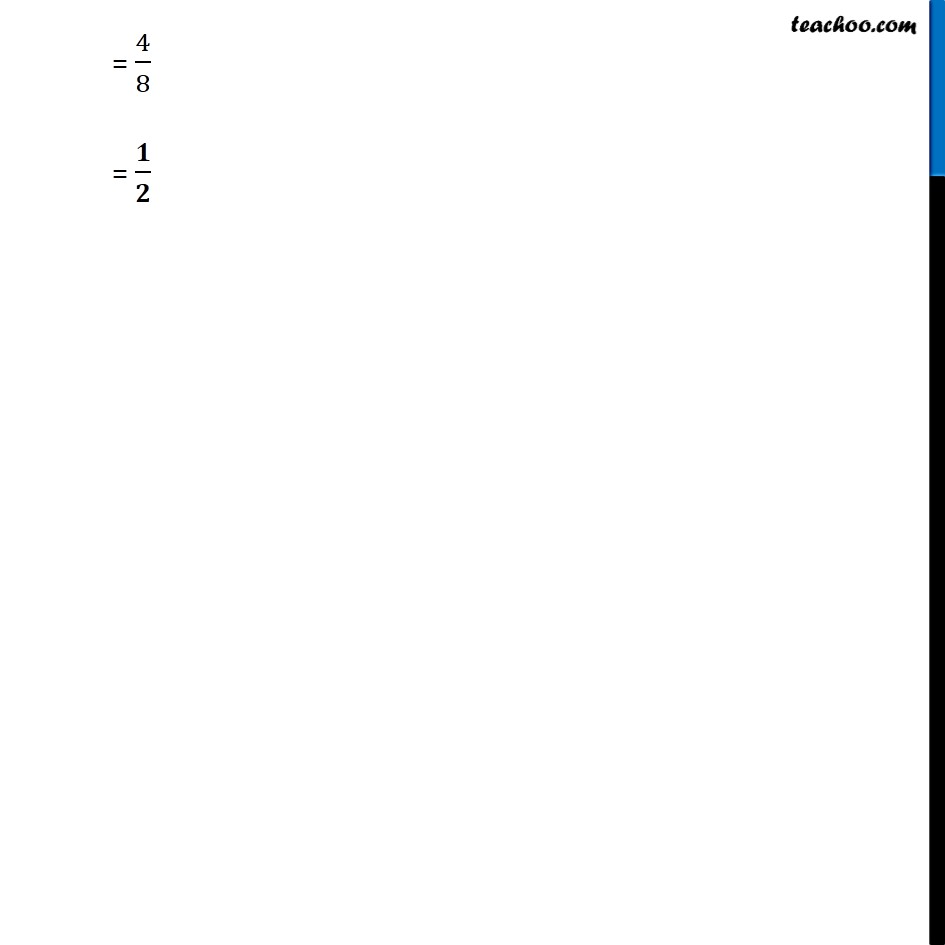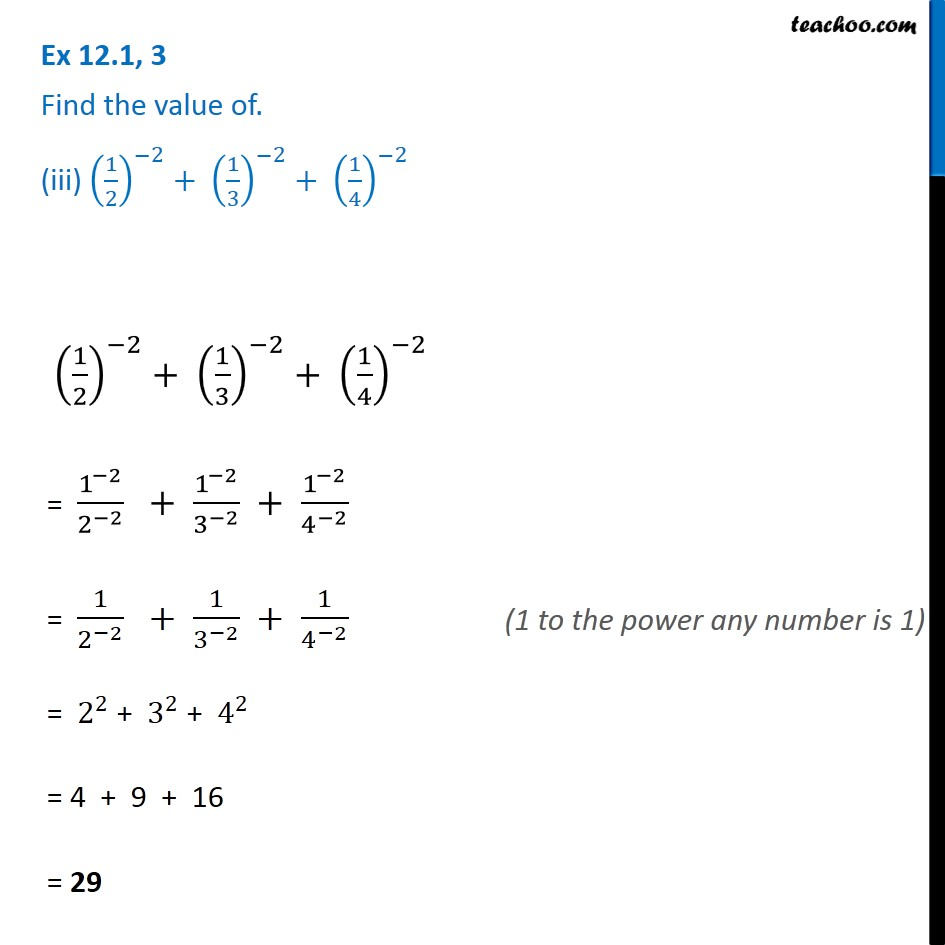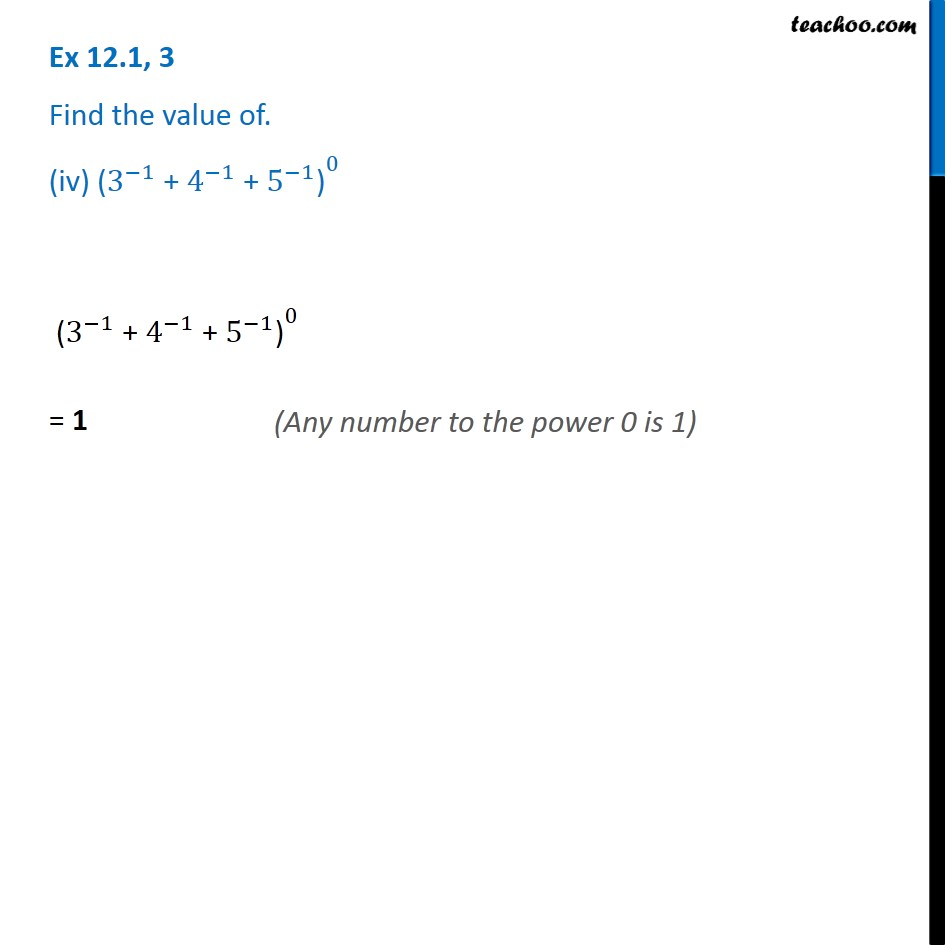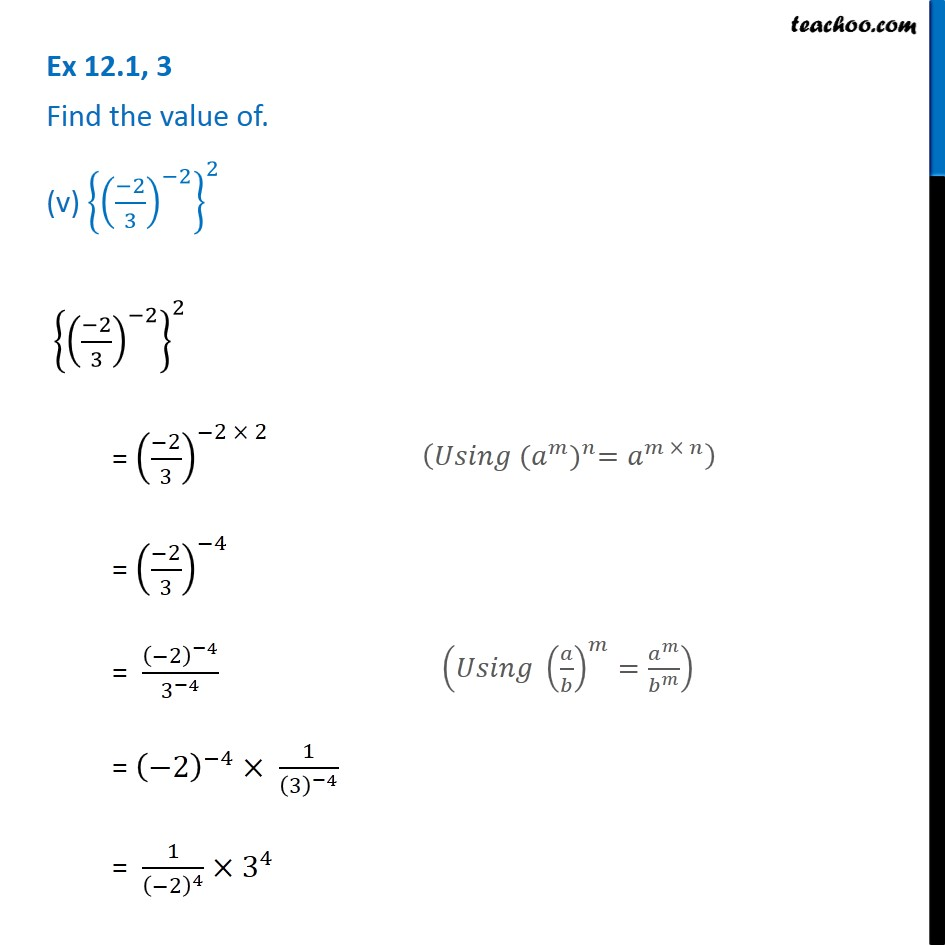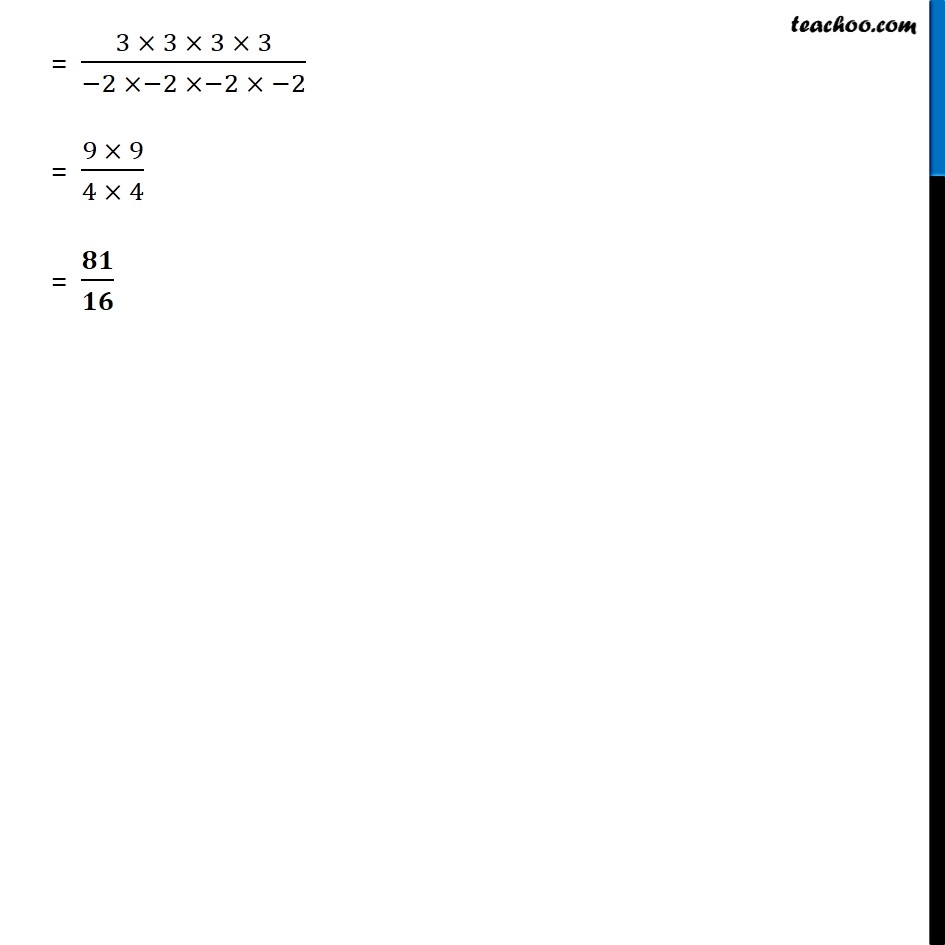1. Chapter 12 Class 8 Exponents and Powers
2. Serial order wise
3. Ex 12.1

Transcript

Ex 12.1, 3 Find the value of. (i) (3^0 + 4^(−1)) × 2^2 (3^0+ 4^(−1)) × 2^2 = (1+4^(−1) )×2^2 = (1+ 1/4)×2^2 = ((4 + 1)/4)×2^2 = 5/4×2^2 = 5/4×4 = 𝟓 (Any number to the power 0 is 1) Ex 12.1, 3 Find the value of. (ii) (2^(−1)× 4^(−1)) ÷〖 2〗^(−2) (2^(−1)× 4^(−1)) ÷〖 2〗^(−2) = (2×4)^(−1) ÷〖 2〗^(−2) = (8)^(−1) ÷〖 2〗^(−2) = 8^(−1)/2^(−2) = 8^(−1)×1/2^(−2) = 1/8^1 ×2^2 = (2 × 2)/8 (𝑈𝑠𝑖𝑛𝑔 𝑎^𝑚×𝑏^𝑚=〖(𝑎×𝑏)〗^𝑚 ) = 4/8 = 𝟏/𝟐 Ex 12.1, 3 Find the value of. (iii) (1/2)^(−2)+ (1/3)^(−2)+ (1/4)^(−2) (1/2)^(−2)+ (1/3)^(−2)+ (1/4)^(−2) = 1^(−2)/2^(−2) + 1^(−2)/3^(−2) + 1^(−2)/4^(−2) = 1/2^(−2) + 1/3^(−2) + 1/4^(−2) = 2^2 + 3^2 + 4^2 = 4 + 9 + 16 = 29 (1 to the power any number is 1) Ex 12.1, 3 Find the value of. (iv) 〖"(" 3^(−1) " + " 4^(−1) " + " 5^(−1) ")" 〗^0 〖"(" 3^(−1) " + " 4^(−1) " + " 5^(−1) ")" 〗^0 = 1 (Any number to the power 0 is 1) Ex 12.1, 3 Find the value of. (v) {((−2)/3)^(−2) }^2 {((−2)/3)^(−2) }^2 = ((−2)/3)^(−2 × 2) = ((−2)/3)^(−4) = (−2)^(−4)/3^(−4) = (−2)^(−4)× 1/(3)^(−4) = 1/(−2)^4 ×3^4 (𝑈𝑠𝑖𝑛𝑔 〖(𝑎^𝑚)〗^𝑛=𝑎^(𝑚 × 𝑛) ) (𝑈𝑠𝑖𝑛𝑔 (𝑎/𝑏)^𝑚=𝑎^𝑚/𝑏^𝑚 ) = (3 × 3 × 3 × 3)/(−2 ×−2 ×−2 × −2) = (9 × 9)/(4 × 4) = 𝟖𝟏/𝟏𝟔

Ex 12.1